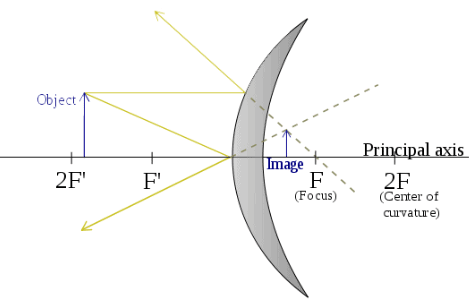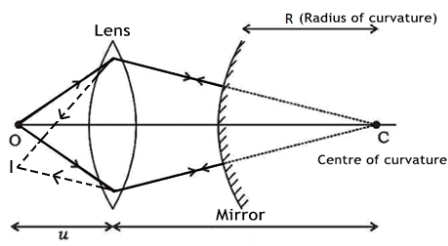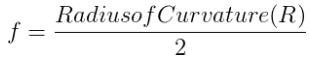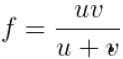Courses

# Theory & Procedure, Convex Mirror (Focal Length) Class 12 Notes | EduRev

## JEE : Theory & Procedure, Convex Mirror (Focal Length) Class 12 Notes | EduRev

The document Theory & Procedure, Convex Mirror (Focal Length) Class 12 Notes | EduRev is a part of the JEE Course Physics Class 12.
All you need of JEE at this link: JEE

Our Objective:

To find the focal length of a convex mirror using a convex lens.

The Theory:

Convex mirror

A convex mirror is a curved mirror in which the reflecting surface bulges towards the light source. Convex mirrors reflect light outwards; therefore they are not used to focus light. A convex mirror is also known as fish eye mirror or diverging mirror.
The image formed by a convex lens is virtual and erect, since the focal point (F) and the centre of curvature (2F) are both imaginary points "inside" the mirror that cannot be reached. As a result, images formed by these mirrors cannot be projected on a screen, since the image is inside the mirror. Therefore, its focal length cannot be determined directly. The image is smaller than the object, but gets larger as the object approaches the mirror. The ray diagram of a convex mirror is shown below.The focal length of a convex mirror can be determined by introducing a convex lens between the object and the convex mirror. An image can be obtained with the help of a convex lens side by side with the object when the convex mirror reflects the rays along the same path, i.e., when the rays fall normally on the mirror. Then, the radius of curvature, R, of the mirror is the distance between the screen and the mirror.The focal length f of the convex mirror is calculated using the formula,Learning Outcomes:

Students understand the following terms:

• Convex mirror
• Focal point

Students are better able to do the experiment in the real laboratory once they understand the procedure.

Materials Required

• Concave mirror
• Stand
• Screen
• Illuminated wire gauze.
• Metre scale

Real lab Procedure

By distant object method :

• Fix the given concave mirror on the stand and place it on a table, facing towards a distant object.
• Arrange the screen on the table so that the image of the distant object is obtained on it.
• Measure the distance between mirror and screen using a metre scale. It can be taken as the focal length (f) of the mirror.

By u-v method :

• Using the focal length obtained by distant object method set the values of u (distance between mirror and object) ranging from 1.5f to 2.5f. Divide the range into a number of equal steps.
• Place the mirror in front of an illuminated wire gauze. It acts as the object.
• Now, fix the mirror at the distance u (which is obtained as 1.5f) from the wire gauze.
• Place the screen on the table facing the mirror in such a way that the reflected image lies on the screen.
• We can adjust the position of the screen to get the clear image of the wire gauze.
• Keeping the distance between object and mirror fixed, adjust the position of screen in order to get the clear image of the object.
• Measure the distance between mirror and wire gauze, as well as mirror and screen. Take these values as u and v respectively.
• Record the values of u and v in a tabular column.
• Calculate the focal length of the given concave mirror by using the relation, f = uv/(u+v).
• Repeat the experiment for different values of u (up to 2.5f) and in each time, measure v and record it in the tabular column.
• Calculate the focal length (f) of the concave mirror each time.
• Calculate the mean of all focal lengths to get the correct focal length of the given concave mirror.
• The focal length of the mirror can also be measured graphically by plotting graphs between u & v, and 1/u & 1/v.
• We can repeat the experiment with concave mirrors of different focal lengths.

Simulator Procedure (as performed through the Online Labs)

• Select the focal length using the slider.
• Click on the ‘Light On/Off’ button to turn On/Off the wire gauze.
• Select the distance between the mirror and the object (u) using the slider.
• Change the distance between the mirror and the screen (v) suing the slider to get a clear image of the wire gauze on the screen.
• You can calculate the focal length of the mirror using the equation,• You can verify your result by clicking on the ‘Show result’ button.
• To redo the experiment, click the ‘Reset’ button.
Offer running on EduRev: Apply code STAYHOME200 to get INR 200 off on our premium plan EduRev Infinity!

## Physics Class 12

204 videos|288 docs|125 tests

,

,

,

,

,

,

,

,

,

,

,

,

,

,

,

,

,

,

,

,

,

,

,

,

;